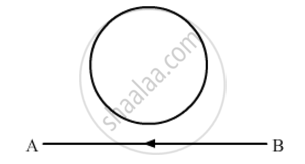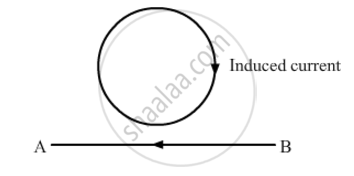# The Electric Current Flowing in a Wire in the Direction from B to a is Decreasing. Find Out the Direction of the Induced Current in the Metallic Loop Kept Above the Wire as Shown. - Physics

The electric current flowing in a wire in the direction from B to A is decreasing. Find out the direction of the induced current in the metallic loop kept above the wire as shown.#### Solution

The decreasing magnetic field in the loop due to the decreasing current in wire AB is into the plane of the paper (perpendicular to the plane). So, the direction of the induced current in the loop will be such that it produces an inward magnetic field (perpendicular to the plane). Thus, the current induced in the loop is in clockwise direction (using the right-hand thumb rule)Concept: Magnetic Force
Is there an error in this question or solution?
2013-2014 (March) All India Set 1

Share